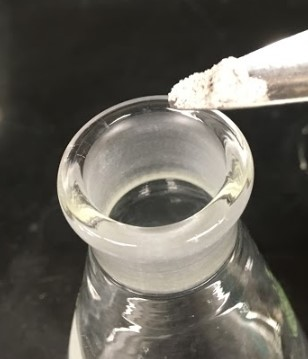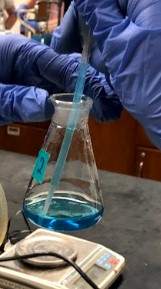$$\newcommand{\vecs}{\overset { \rightharpoonup} {\mathbf{#1}} }$$ $$\newcommand{\vecd}{\overset{-\!-\!\rightharpoonup}{\vphantom{a}\smash {#1}}}$$$$\newcommand{\id}{\mathrm{id}}$$ $$\newcommand{\Span}{\mathrm{span}}$$ $$\newcommand{\kernel}{\mathrm{null}\,}$$ $$\newcommand{\range}{\mathrm{range}\,}$$ $$\newcommand{\RealPart}{\mathrm{Re}}$$ $$\newcommand{\ImaginaryPart}{\mathrm{Im}}$$ $$\newcommand{\Argument}{\mathrm{Arg}}$$ $$\newcommand{\norm}{\| #1 \|}$$ $$\newcommand{\inner}{\langle #1, #2 \rangle}$$ $$\newcommand{\Span}{\mathrm{span}}$$ $$\newcommand{\id}{\mathrm{id}}$$ $$\newcommand{\Span}{\mathrm{span}}$$ $$\newcommand{\kernel}{\mathrm{null}\,}$$ $$\newcommand{\range}{\mathrm{range}\,}$$ $$\newcommand{\RealPart}{\mathrm{Re}}$$ $$\newcommand{\ImaginaryPart}{\mathrm{Im}}$$ $$\newcommand{\Argument}{\mathrm{Arg}}$$ $$\newcommand{\norm}{\| #1 \|}$$ $$\newcommand{\inner}{\langle #1, #2 \rangle}$$ $$\newcommand{\Span}{\mathrm{span}}$$$$\newcommand{\AA}{\unicode[.8,0]{x212B}}$$

Objectives

• To determine the reduction potential ranges for vanadium (IV), (III), and (II) based on color changes of each oxidation state.

## Introduction

Transition metals with electrons in the d orbital are able to form colored complexes based on their oxidation state. Vanadium is one such metal. It is capable of oxidation states of +2, +3, +4, and +5. Each of these states have a different color: purple (II), green (III), blue (IV) and yellow (V). In addition to different colors, the reductive potential is also different for each state. However, the potentials are generally not published in textbook tables of standard reductive potentials. Through the use of chemicals that are given in standard tables, you will be able to determine the potential for the three reactions given below.

$\ce{VO_{2}^{+}}(aq) + 2\ce{H^{+}}(aq) + \ce{e^{-}} \ce{<=>} \ce{VO^{2+}}(aq) + \ce{H_{2}O}(l) \label{1}$

$\ce{VO^{2+}}(aq) + 2\ce{H^{2}}(aq) + \ce{e^{-}} \ce{<=>} \ce{V^{3+}}(aq) + \ce{H_{2}O}(l) \label{2}$

$\ce{V^{3+}}(aq) + \ce{e^{-}} \ce{<=>} \ce{V^{2+}}(aq) \label{3}$

Vanadium (V) is prepared using Ammonium vanadate $$\ce{NH_{4}VO_{3}}$$. In acidic conditions, $$\ce{VO_{3}^{-}}$$ is converted to $$\ce{VO_{2}^{+}}$$.

$\ce{VO_{3}^{+}}(aq) + 2\ce{H^{+}}(aq) \ce{<=>} \ce{VO_{2}^{+}}(aq) + \ce{H_{2}O} \label{4}$

Both of these species have vanadium in the +5 oxidation state, resulting in a yellow solution. When reacting with a strong enough reducing agent, vanadium will transition from $$\ce{VO_{2}^{+}}$$ (V) to $$\ce{VO^{2+}}$$ (IV) to $$\ce{V^{3+}}$$ (III) and finally to $$\ce{V^{2+}}$$ (II). You will see the transition for each of these oxidation states show up as a different color. From the yellow color of $$\ce{VO_{2}^{+}}$$, you will see a blue solution of $$\ce{VO^{2+}}$$ form. The solution will then turn a shade of green because of $$\ce{V^{3+}}$$. Finally, with the addition of heat and stirring, you should be able to obtain a lavender purple solution from $$\ce{V^{2+}}$$. Note however, the transition from yellow to blue will look green during the transition. Do not mistake this for another oxidative state. It is simply the mixing of the blue and yellow ions to give a green appearance.

Not all reducing agents are created equal. Some are only able to reduce the vanadium to the +4 and +3 state or possibly only to the +4 state. It all depends on the standard reduction potential of the specific chemical being used. In this lab, we will be employing zinc, tin, and silver metals to reduce vanadium. Based on your results and the standard reduction potential of each addition, you should be able to determine an approximate range for each of the standard reduction potentials for equations 1-3.

Pre-Lab Assignment

• Look up the standard reductive potentials for the following reactions.
• $$\ce{Zn^{2+}} + 2\ce{e^{-}} \ce{<=>} \ce{Zn}\label{5}$$
• $$\ce{Sn^{2+}} + 2\ce{e^{-}} \ce{<=>} \ce{Sn} \label{6}$$
• $$\ce{Ag^{+}} + 2\ce{e^{-}} \ce{<=>} \ce{Ag} \label{7}$$
• $$\ce{NO_{3}^{2+}} +3\ce{H^{+}} + 2\ce{e^{-}} \ce{<=>} \ce{HNO_{2}} \label{8}$$
• $$\ce{Br_{2}} + 2\ce{e^{-}} \ce{<=>} \ce{Br_{2}} \label{9}$$
• $$\ce{ClO_{3}^{-}} +6\ce{H^{+}} + 6\ce{e^{-}} \ce{<=>} \ce{Cl^{-}} + 3\ce{H_{2}O} \label{10}$$

## Procedure

Safety and Waste Disposal

• The solutions must be disposed of in the hazardous waste container.
###### Step 1Obtain and label 3 50 mL Erlenmeyer flasks. Please feel free to take pictures along the way. This will help in your documentation of the colors. However, this is not a substitute for good record keeping in your laboratory notebook.

###### Step 2Place 10 mL of the yellow vanadium (V) solution in each of the 50 mL flasks.

###### Step 3To flask number one, add a small amount of zinc dust.

###### Step 5

Finally, to flask number 3, add a small amount of tin dust.

###### Step 6

Stopper each flask with a small cotton ball.

###### Step 7

Watch each of the three flasks for color changes. Note, the first color change will be from yellow to blue. However, you will get a green intermediate. Why?

###### Step 8To speed the reaction, you can place the flask on a hot plate set to low heat. Be sure to add a stir bar and stir while the reaction is heating.

###### Step 9

Each flask will end up at a different oxidation state and thus a different color. You will eventually obtain a blue solution, a green solution (different than the green solution in step 7), and a lavender solution. If you do not end up with four different colors, add a small amount of additional metal to the appropriate flasks. This is particularly true of the lavender solution.

###### Step 10Once you reach the final oxidation states/colors, take 1 mL portions and add it to four test labeled test tubes. You will eventually have 16 test tubes total (4 yellow, 4 blue, 4 green, and 4 lavender). Be sure not to draw up any solid metal that may be left in the bottom of the flask.

###### Step 11

To the lavender set of test tubes, add 10 drops of the following compounds: HNO3, KNO2, NaBr, and NaClO3, one compound per test tube. Repeat for the other 3 oxidation states. Be sure to begin with the lavender set though, as it will change back to green if allowed to sit long enough.

###### Step 12

Record the color changes that you see after each of the additions.

## Observations

Test Observation Summary of Reaction
Ammonium vanadate + Acid (The first reactions that took place when the solution was created) White solid dissolved in an acidic solution to produce a yellow solution

5+ $$\ce{->}$$ 5+

$$\ce{VO_{3}^{-}} \ce{->} \ce{VO_{2}^{+}}$$

Test Observation (Include original solution color and final solution color) Summary of Reaction

Vanadium (V) + $$\ce{HNO_{3}}$$

Vanadium (IV) + $$\ce{HNO_{3}}$$

Vanadium (III) + $$\ce{HNO_{3}}$$

Vanadium (II) + $$\ce{HNO_{3}}$$

Vanadium (V) + $$\ce{KNO_{2}}$$

Vanadium (IV) + $$\ce{KNO_{2}}$$

Vanadium (III) + $$\ce{KNO_{2}}$$

Vanadium (II) + $$\ce{KNO_{2}}$$

Vanadium (V) + $$\ce{NaBr}$$

Vanadium (IV) + $$\ce{NaBr}$$

Vanadium (III) + $$\ce{NaBr}$$

Vanadium (II) + $$\ce{NaBr}$$

Vanadium (V) + $$\ce{NaClO_{3}}$$

Vanadium (IV) + $$\ce{NaClO_{3}}$$

Vanadium (III) + $$\ce{NaClO_{3}}$$

Vanadium (II) + $$\ce{NaClO_{3}}$$

## Data Processing

Based on the reductive potential values that you looked up for the prelab, determine the range of the potentials for each of the vanadium oxidation state changes.

V(V) → V(IV)      $$\ce{VO_{2}^{+}}(aq) + 2\ce{H^{+}}(aq) + \ce{e^{-}} \ce{<=>} \ce{VO^{2+}}(aq) + \ce{H_{2}O}(l)$$        Ered range

V(IV) → V(III)    $$\ce{VO^{2+}}(aq) + 2\ce{H^{2}}(aq) + \ce{e^{-}} \ce{<=>} \ce{V^{3+}}(aq) + \ce{H_{2}O}(l)$$          Ered range

V(III) → V(II)     $$\ce{V^{3+}}(aq) + \ce{e^{-}} \ce{<=>} \ce{V^{2+}}(aq)$$                                           Ered range

8: Vanadium Rainbow is shared under a not declared license and was authored, remixed, and/or curated by LibreTexts.